Contents >> Applied Mathematics >> Numerical Methods >> Algebraic and Transcendental Equations >> Introduction

 Algebraic and transcendental equations - Introduction Introduction The finding of exact roots of the algebraic or transcendental equation (that is the equations no algebraic, for example, trigonometric, logarithmic or irrational) is frequently enough difficult problem which is not solved analytically by means of final formulas. Besides sometimes in practice the equation contains factors, which values are given approximately so to speak about the exact solution of the equations in such cases at all has no sense. Therefore problems of the approached definition of roots of the equation and a corresponding estimation of their accuracy have great value and today. The approached methods of the solution of the equations can be divided conditionally on graphic and numerical ones. We’ll consider only numerical methods of the solution. Let's consider the equation: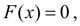(1) where F ( x ) – is a continuous function, defined in the interval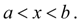In some cases existence and a continuity of the first and second derivatives of this function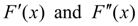is required, that each time will especially stipulate. Any value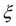at which: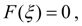(2) is called a root of the equation (1) or zero of function F ( x ). Let's consider, that the equation (1) has only the isolated roots, that is for each root of the equation (1) there is a neighborhood which is not containing other roots of this equation. Process of separation of roots is in detail described [1, 2] and here again is not considered. The approached finding of the isolated real roots is carried out in two stages: 1) Finding of the approached value of a root – so-called zero approach . 2) Précising of the approached value of a root by iterations or consecutive approximations until the given accuracy of the solution will be reached. Let's stop in detail at the second stage as the finding of zero approach is the specific problem solved usually either on the basis of physical reasons or design features, or by the graphic decision of the equation

 < Previous Contents Next >